# 8 Main Types of Costs involved in Cost of Production and Revenue (With Diagram)

The following points highlight the eight main types of costs involved in cost of production and revenue.

The costs are: 1. Real Cost 2. Opportunity Cost 3. Money Cost 4. Production Costs 5. Selling Costs 6. Fixed and Variable Costs 7. Fixed Costs or Supplementary Costs 8. Average and Marginal Cost.

#### Cost Type # 1. Real Cost:

The term “real cost of production” refers to the physical quantities of various factors used in producing a commodity.

In other-words—Real cost signifies the aggregate of real productive resources absorbed in the production of a commodity or a service.

Marshall has described “real cost” as the production of a commodity generally requires many different kinds of labour and the use of capital in many forms.

The exertions of all the different kinds of labour that are directly or indirectly involved in making it, together with the abstinences or rather the waiting’s required for saving the capital used in making it ; all these efforts and sacrifices together will be called the real cost of production of a commodity. Further, he had said that the real cost of production connotes the toil, trouble and sacrifice of factors in producing a commodity.

Thus, the Marshallian concept of real cost has only a philosophical significance. In practice, however it is difficult to measure it. It has little significance in the analysis of price determination although more significance from the social point of view.

The main difficulty with this concept is that the efforts and sacrifices implicit in the real cost of production and purely subjective and cannot be subjective to accurate monetary measurement Prof. Handerson and Quant have observed that “the real cost leads us into the quagmire of unreality and dubious hypothesis.”

#### Cost Type # 2. Opportunity Cost:

The opportunity cost is also known as ‘transfer cost’ or ‘alternate cost’.

Prof. Lipsey has defined it as “the opportunity cost of using any factor is what is currently forgone by using it.”

Whereas Mrs. Joan Robinson has defined opportunity cost in terms of transfer cost. According to her, ‘The price which is necessary to retain a given unit of factor in a certain industry may be called its transfer earning or transfer price.”

The utility of the study of opportunity cost lies in the theory of production. The factor must be paid at least that price which they are able to obtain in the alternate use. If the
opportunity cost in another field is more the labour will shift from one industry to those industries where transfer earning is more. But the main drawback of this concept is that it is not applicable to a specific factor i.e., a factor which can be put to single use. Since the factor is a single use factor, it can have no alternative or opportunity cost.

#### Cost Type # 3. Money Cost:

‘Money Cost’ is the monetary expenditure on inputs of various kinds. It is that total money expenses incurred by a firm in producing a commodity. They include wages and salaries of labour; cost of raw-material, expenditure on machines and equipment, depreciation and obsolescence charges on machines building and other capital goods; rent on building; interest on capital invested and borrowed ; normal profits of business, expenses on power, light, fuel, advertisement and transportation, insurance charges and all types of taxes.

The money cost includes both:

(a) Implicit Costs

(b) Explicit Costs

(a) Explicit Costs:

This includes those payments which are made by the producer to those factors of production which do not belong to the producer himself. These costs are mostly in the nature of contractual payment made by the producer to the owner of those factors whose services were bought by him for the purpose of production, e.g., the payment made for raw- materials, power, light, fuel the wages and salaries paid to the workers and other staff, the rent paid on the land and the interest paid on the borrowed capital etc. Payments on all such accounts will be included in explicit cost.

(b) Implicit Costs:

Implicit costs are also known as imputed costs. These costs arise in the case of those factors which are possessed and supplied by the producer himself. Here we cannot assign exact money value but can term them in imputed values, e.g., a producer may contribute his own building or premises for running the business, his own capital and working also as Managing Director of the firm.

As such he is entitled to get rent on his own premises, interest on capital contributed by him and also salary for his work as Managing Director. All these items will be included in the implicit costs.

Difference between the Explicit and Implicit Costs:

1. Difference between the two is that the former can be measured in terms of money value, whereas the latter is in terms of imputed values.

2. The former is contractual payment whereas the second is the price paid to himself.

#### Cost Type # 4. Production Costs:

Production costs have been called as the total amount of money spent in the production of goods. They include the cost of raw materials and freight thereon, the costs of manufacture, i.e., the wages of workers engaged in the manufacture of the commodity and salaries of the manager and other office staff including those of peons etc.

They also include and cover other overheads expenses like—rent, interest on capital, taxes, insurance and other incidental expenses like—cost of repairs and replacements. They include both prime costs and supplementary costs.

#### Cost Type # 5. Selling Costs:

Selling costs are the costs of marketing, advertisement and salesman­ship. These costs are incurred to attract customers, expand market and capture more business and retain the existing business. These costs are the essential costs of the competitive economy.

They are especially important in the case of imperfect competition in which goods are not identical but substitutes. The manufacturers resort to what is called product differentiation in order to change the demand curve of a particular seller to his advantage.

Selling costs are an important aspect of an imperfect market and have no place in a fully competitive market where the dealers are supposed to be fully aware of the quality of the goods and the conditions of the market.

#### Cost Type # 6. Fixed and Variable Costs:

Cost refer to the prices paid to the factors of production, we find prices paid to fixed factors, and the prices paid to the variable factors which are termed as the fixed costs and the variable costs respectively. Thus, the cost of production of a commodity is composed of two types of costs, i.e., Variable Costs and Fixed Costs, also called Prime and Supplementary Costs respectively.

#### Cost Type # 7. Fixed Costs or Supplementary Costs:

Fixed Costs or Supplementary Costs are the amount spent by the firm on fixed inputs in the short-run. Fixed costs are those costs which remain constant, irrespective of the level of output. These costs remain unchanged even if the output of the firm is nil. Fixed costs, therefore, are known as “Supplementary Costs” or “Overhead Costs”. Fixed Costs, in the short-run, remain fixed because the firm does not change its size and the amount of fixed factors employed.

Fixed or Supplementary Costs usually include:

1. Payments of rent for the building,

2. Interest paid on capital,

4. Depreciation and maintenance allowances,

5. Administrative expenses—Salaries of managerial and office staff etc.,

6. Property and business taxes, licence fees etc.

These costs are overhead costs in the sense that they are to be incurred even if the firm is closed down temporarily and the current production is nil. Further, they do not change as the output increases. Thus, fixed costs are also referred to as “Unavoidable Contractual Costs” which occur even if there is no output. In brief, the costs incurred on the business plant are called fixed costs.

#### Cost Type # 8. Average and Marginal Cost:

We have examined earlier that what is the total cost i.e., Fixed Cost + Variable Cost taken together is called Fixed Total Cost. Now, we are going to discuss what is Average and Marginal Costs? Average Cost is also known as cost per unit. If total cost of production is divided by the total number of units produced, we get the average cost.

In other-words, Average Cost at any output = Total Cost/ Units of Output

Average cost is the sum of average variable cost and average fixed cost. It is also called average total cost. If the total cost of producing 120 units of a commodity is 2400 rupees then average cost will be 2400/ 120 = Rs. 20. On the other-hand, Average Cost helps in fixing or determining the total quantity for sale. The difference between average revenue and average cost shows the profit per unit. If the profit is multiplied with the total production (Units of production) we get the total or gross profit.

Marginal Cost:

Marginal Cost is the cost of producing and additional unit of output. In other-words, marginal cost is the addition made to the total cost by producing one more unit of output.

For Example:

If the total cost of producing 120 units is 2400 rupees and the total cost of producing 121 units is 2436 rupees, the marginal cost in this case will be equal to 36 rupees, (2436-2400).

The concepts of total cost, average cost and marginal cost can be understand easily from the following table:

In the above table, marginal cost of the second is found out by subtracting Rs. 60 from Rs. 80 (80 – 60 = 20). Marginal Costs of subsequent units are obtained in the same manner. The marginal cost is the addition made to the total cost at each step.

Relationship between Average Cost and Marginal Cost:

It should be noted that average and marginal cost are related to each other. It means when average cost curve falls, the marginal cost falls sharply. In the figure upto K point, but marginal cost falls more sharply. When average cost is minimum marginal cost intersect it at that point (at K). At K the average cost is minimum and marginal cost intersect it.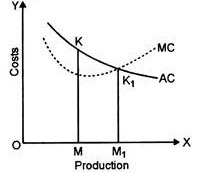When average cost increases marginal cost increases more sharply. As is shown in the diagram. After K point the average cost rises but marginal cost rises more sharply. It is also clear that average cost is still falling. Whereas the marginal cost begins to rise.

In the above diagram, after point K, the average cost is still falling, but the marginal cost is rising. It means the increase in the total cost affects the average cost after being distributed among units of production. But in case of marginal cost, it shows an increase in only one unit, which can be shown by the above diagram.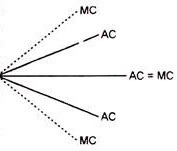Marginal cost reaches its lowest point sooner than the average cost at an unexpectedly smaller level of production. The above relationship between Average Cost and Marginal Cost can be explained with the help of a diagram. Read this diagram anti-clock-wise in the beginning when Average Cost is falling Marginal Cost lies below it. When AC is constant MC is also constant, when AC is rising MC rises above it. But this is applicable only in a crude way.

There are certain exceptions to the above usual relationship between AC and MC:

I. When AC falls but MC rises:

In this case the situation is generally available when the AC is moving towards the lowest average cost situation. The addition made in the total cost by the production of an additional unit of production increases i.e., MC increases but the addition is less effective to AC as shown in the following table given: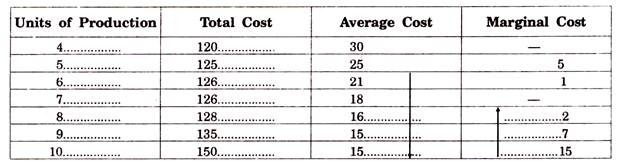After the production of 7th unit the AC is falling whereas MC has started increasing. Thus, we can say that when AC falls MC can rise.

II. When AC rises but MC falls:

This situation arises when the production is being conducted under the increasing costs. After attaining a minimum average cost, with the additional production Average Cost increases.

In this case Marginal Cost is always more than Average Cost as shown in the following schedule: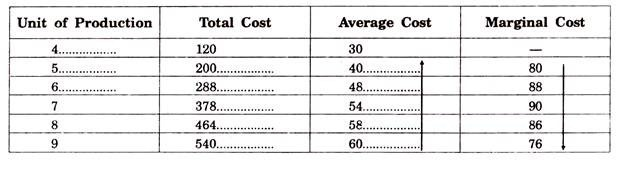The average cost is increasing with the additional output, but marginal cost is increasing upto 7th unit but after that decreasing. Thus, we can see that when Average Cost is rising, Marginal Cost can fall.

III. When Average Cost falls but Marginal Cost remains constant:

This excep­tional situation can arise when the total cost is composed of fixed costs and variable costs in such a way that in the short period total fixed cost do not undergo any change and only a given variable cost is required to produce more and that too, at a constant rate as shown in the following table: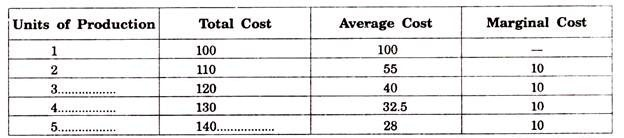The above exceptions to the usual relationship between Average Cost and Marginal Cost of little significance. In the theory of value, we use only five relationships as discussed.

Significance of Average Cost and Marginal Cost in the Theory of Value:

Average Cost and Marginal Cost along with the Average and Marginal Revenue help the firm to achieve an equilibrium. Under the conditions of perfect Competition Average Revenue and Marginal Revenue coincide with each other and their curves are parallel to X-axis. Average Cost and Marginal Cost determines the level of output. Marginal Cost is helpful in determining the level of output whereas Average Cost determines the profits and losses of the firm.

In the long period, firm attain equilibrium where Average Cost = Marginal Cost = Average Revenue = Marginal Revenue. But in monopoly, the Average Revenue and Marginal Revenue Curves are downwards sloping curves. The output is determined where Marginal Cost is equal to Marginal Revenue but afterwards Marginal Cost must be rising. The profits and losses are calculated by the difference between Average Revenue and Average Cost. Thus, both Average Cost and Marginal Cost plays a vital role in determining the prices of the product.